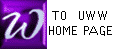THE INVERSE OF AN n x n MATRIX

 See our text ( Rolf, Page 163) for a discussion of matrix inverses. Briefly, matrix inverses behave as reciprocals do for real numbers : the product of a matrix and it's inverse is an identity matrix.

Let A be the name of our nxn matrix: non-square matrices have no inverse. The following steps will produce the inverse of A, written A-1. Note the similarity between this method and GAUSS/JORDAN method, used to solve a system of equations.


 Augment the nxn matrix A with the nxn identity matrix In ; see Text ( Rolf, Pg 163) or scroll below [ A | In ]

 Pivot on matrix elements in positions 1-1, 2-2, 3-3, continuing through n-n in that order, with the goal of creating a copy of the identity matrix In in the left portion of the augmented matrix. If one of the pivoting elements is zero, then first interchange it's row with a lower row. If no such interchange produces a non-zero pivot element, then the matrix A has no inverse. This step  is equivalent to step 2 on Pg 163 of our text Rolf, resulting in (REDUCED) DIAGONAL FORM. See an example below, and try the Pivot Engine when you check your pivoting skills. [ A | In ] ===> [ In | A-1 ]

 When step  above is done, the right half of the latest augmented matrix will be the desired inverse, A-1; write it separately, and you're done, as in the example below.

 Note 1 : Professor McFarland names row operations just a bit differently from our text: follow Prof McFarland's naming style.

Note 2 : Check out Prof McFarland's interactivePIVOT ENGINE as you use row operations.

Note 3 : Compare the above 3 steps for those used in GAUSS/JORDAN.

EXAMPLE OF FINDING THE INVERSE OF A MATRIX A

See our text (Rolf, Pg 163) for one example; below is another example :

We must find the inverse of the matrix A at the right
 A =1 2-2 -1 1-2 321Below is the same matrix A, augmented by the 3x3 identity matrix. The first pivot encicled in red
Below are the row operations required for the first pivoting
 Next pivot on "3" in the 2-2 position below, encircled in red
 The columns of the 3x3 identity matrix are colored blue as they re-appear on the left side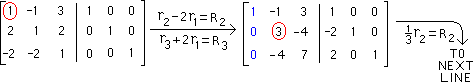Below is the result of performing P1, so the pivot (2-2 position) is now "1". Next we perform P2
Row operations
of P2
are below
 The result of the second pivoting is below. We now pivot on the element in the 3-3 position, encircled in red below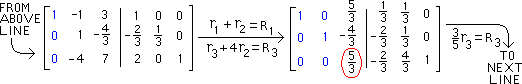Below is the result of performing P1, so the pivot (3-3 position) is now "1". Next we perform P2.
Below are the row operations of P2
 The result of the third (and last) pivoting is below with 3x3 identity matrix in blue
 The matrix below is NOT A-1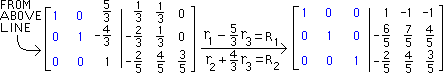(REDUCED)DIAGONALFORM E

Thus, our final step is to
separate the desired inverse
from the above matrix:

 A-1 =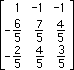Note : THE MATRIX INVERSE METHOD for solving a system of equations will use the above discussion, and even continue the above problem.